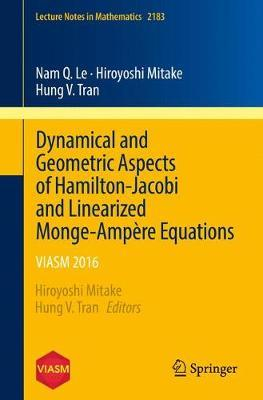# Dynamical and Geometric Aspects of Hamilton-Jacobi and Linearized Monge-Ampere Equations: VIASM 2016

## Hiroyoshi Mitake, Hung V. Tran, Nam Le• Format
• Publisher
• Country
• Published
• Pages
• ISBN

# Dynamical and Geometric Aspects of Hamilton-Jacobi and Linearized Monge-Ampere Equations: VIASM 2016

## Hiroyoshi Mitake, Hung V. Tran, Nam Le

Consisting of two parts, the first part of this volume is an essentially self-contained exposition of the geometric aspects of local and global regularity theory for the Monge-Ampere and linearized Monge-Ampere equations. As an application, we solve the second boundary value problem of the prescribed affine mean curvature equation, which can be viewed as a coupling of the latter two equations. Of interest in its own right, the linearized Monge-Ampere equation also has deep connections and applications in analysis, fluid mechanics and geometry, including the semi-geostrophic equations in atmospheric flows, the affine maximal surface equation in affine geometry and the problem of finding Kahler metrics of constant scalar curvature in complex geometry. Among other topics, the second part provides a thorough exposition of the large time behavior and discounted approximation of Hamilton-Jacobi equations, which have received much attention in the last two decades, and a new approach to the subject, the nonlinear adjoint method, is introduced. The appendix offers a short introduction to the theory of viscosity solutions of first-order Hamilton-Jacobi equations.

This item is not currently in-stock. It can be ordered online and is expected to ship in 7-14 days

Our stock data is updated overnight, and availability may change throughout the day for in-demand items. Please call the relevant shop for the most current stock information. Prices are subject to change without notice.